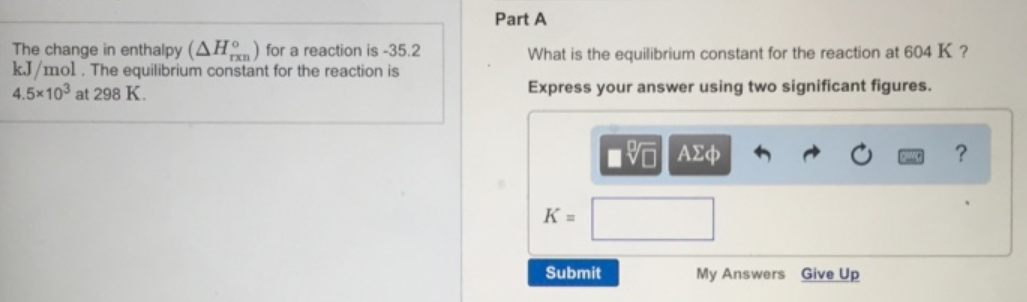# Problem: The change in enthalpy (ΔH° rxn) for a reaction is -35.2 kJ/mol. The equilibrium constant for the reaction is 4.5x103 at 298 K. What is the equilibrium constant for the reaction at 604 K? Express your answer using two significant figures.

###### FREE Expert Solution
81% (121 ratings)###### Problem Details

The change in enthalpy (ΔH° rxn) for a reaction is -35.2 kJ/mol. The equilibrium constant for the reaction is 4.5x103 at 298 K. What is the equilibrium constant for the reaction at 604 K? Express your answer using two significant figures.